Courses

# First Law Applied To Flow Process

## 10 Questions MCQ Test Topicwise Question Bank for GATE Mechanical Engineering | First Law Applied To Flow Process

Description
This mock test of First Law Applied To Flow Process for Mechanical Engineering helps you for every Mechanical Engineering entrance exam. This contains 10 Multiple Choice Questions for Mechanical Engineering First Law Applied To Flow Process (mcq) to study with solutions a complete question bank. The solved questions answers in this First Law Applied To Flow Process quiz give you a good mix of easy questions and tough questions. Mechanical Engineering students definitely take this First Law Applied To Flow Process exercise for a better result in the exam. You can find other First Law Applied To Flow Process extra questions, long questions & short questions for Mechanical Engineering on EduRev as well by searching above.
QUESTION: 1

### Neglecting changes in potential and kinetic energies, the shaft work during a steady flow process is given by

Solution:

Work in steady flow process  =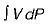Work in non-flow process =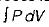QUESTION: 2

### Consider the following statements about steady flow process: 1. The rate of flow of mass energy across the control surface are constant. 2. Thermodynamic properties vary along space as well as time coordinates. 3. Any thermodynamic property will have a fixed value at a particular location and will not alter with time. Which of the above are correct?

Solution:

Thermodynamic properties may vary along space coordinates but do not vary with time.

QUESTION: 3

### Consider following comparative points of S.F.E.E with Bernoulli's equation: 1. These two have several terms in common. 2. Both Bernoulli’s equation and S.F.E.E is valid for viscous compressible fluids. 3. The Bernoulli’s equation is a special limiting case of S.F.E.E Which of the above is/are correct?

Solution:

Bernoulli’s equation valid for frictionless incompressible fluids. S.F.E.E. valid for viscous compressible fluids.

QUESTION: 4

Neglecting changes in kinetic and potential energies, the identity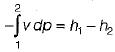for the shaft work during a steady flow process is valid for

Solution:
QUESTION: 5

The term Δh in a control volume equation Q - W = Δh

Solution:

S.F.E.E: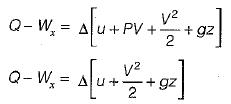Δh accounts for internal energy and pressure forces

QUESTION: 6

A key concept in analyzing the filling of an evacuated tank is

Solution:
QUESTION: 7

Match List-I (Devices) with List-ll (Thermodynamics equations) and select the correct answer using the codes given below the lists: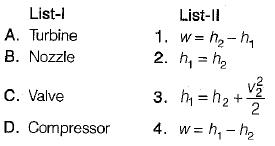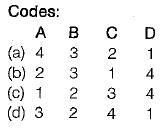Solution:
QUESTION: 8

If steam is throttled its

Solution:

If steam is throttled , its enthalpy remains constant and pressure drop takes place

QUESTION: 9

Which one of the following expression is correct for reversible work done by the system (steady flow) between state 1 and 2 ?

Solution:
QUESTION: 10

Compressed air coming out from a punctured football

Solution: